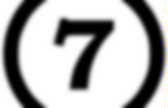Expressions and Equations

Khan Academy - Manipulating Expressions. Khan Academy - Variables and Expressions. Khan Academy - Solving simple equations. Two Step Equation Game. Write variable expressions to represent word problems. Algebraic Expressions Millionaire. Solve 1-Step Equations. Soccer Math - One-Step Equations Game. Model Algebraic Equations with a Balance Scale.